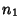## Total Intersection Theorem

If one part of the total intersection group of a curve of orderwith a curve of orderconstitutes the total intersection with a curve of order, then the other part will constitute the total intersection with a curve of order.

References

Coolidge, J. L. A Treatise on Algebraic Plane Curves. New York: Dover, p. 32, 1959.